PSABM6V9 - Student Facing Task---Acc7.5 Lesson 9 Slopes and Equations for All Kinds of Lines (7.G.A, 8.EE.B, 8.EE.B.6)

Which one doesn’t belong?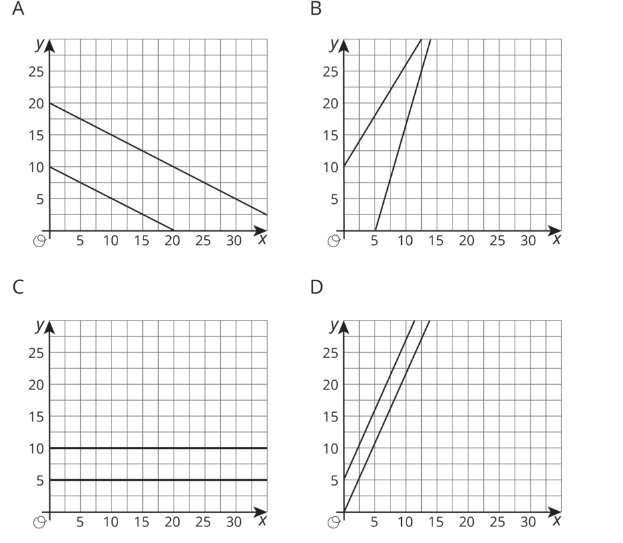Part A)

Plot the points (1,11) and (8,2), and use a ruler to draw the line that passes through them.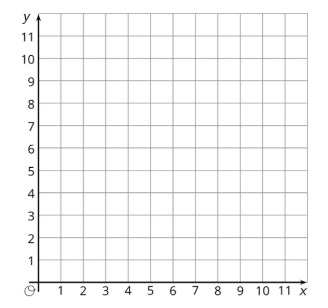Draw your graph on paper, take a picture, and upload it using the image upload icon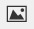If you do not have the ability to upload an image of your work, type "Graph is on paper."

Part B)

Without calculating, do you expect the slope of the line through (1,11) and (8,2) to be positive or negative?

Select one:
Part C)

How can you tell?

Part D)

Calculate the slope of this line.

Find the value of k so that the line passing through each pair of points has the given slope.

(k,2) and (11,14), slope = 2

Type your answer below as a number (example: 5, 3.1, 4 1/2, or 3/2):

Find the value of k so that the line passing through each pair of points has the given slope.

(1,k) and (4,1), slope = -2

Type your answer below as a number (example: 5, 3.1, 4 1/2, or 3/2):

Find the value of k so that the line passing through each pair of points has the given slope.

(3,5) and (k,9), slope =Type your answer below as a number (example: 5, 3.1, 4 1/2, or 3/2):

Find the value of k so that the line passing through each pair of points has the given slope.

(-1,4) and (-3,k), slope =Type your answer below as a number (example: 5, 3.1, 4 1/2, or 3/2):

Find the value of k so that the line passing through each pair of points has the given slope.and, slope = 0

Part A)

Plot at least 10 points whose y-coordinate is -4.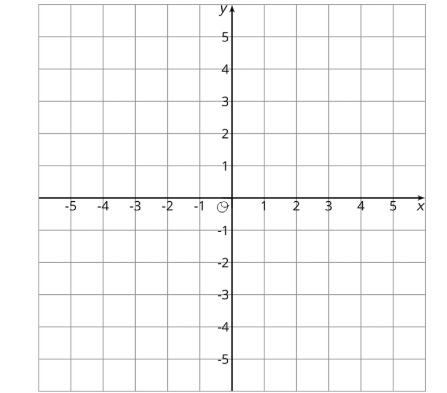Draw your graph on paper, take a picture, and upload it using the image upload iconIf you do not have the ability to upload an image of your work, type "Graph is on paper."

Part B)

What do you notice about the points?

Part C)

Which equation makes the most sense to represent all of the points with y-coordinate?

Select one:
Part D)

Which equation makes the most sense to represent all of the points with y-coordinate?

Explain how you know.

Part E)

Plot at least 10 points whose x-coordinate is 3.

Draw your graph on paper, take a picture, and upload it using the image upload iconIf you do not have the ability to upload an image of your work, type "Graph is on paper."

Part F)

What do you notice about the points?

Part G)

Which equation makes the most sense to represent all of the points with x-coordinate 3?

Select one:
Part H)

Which equation makes the most sense to represent all of the points with x-coordinate 3?

Explain how you know.

Part I)

Graph the equation x = -2.

Draw your graph on paper, take a picture, and upload it using the image upload iconIf you do not have the ability to upload an image of your work, type "Graph is on paper."

Part J)

Graph the equation y = 5.

Draw your graph on paper, take a picture, and upload it using the image upload icon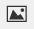If you do not have the ability to upload an image of your work, type "Graph is on paper."

Part A)

Draw the rectangle with vertices (2,1), (5,1), (5,3), (2,3).

Draw your rectangle on paper, take a picture, and upload it using the image upload iconIf you do not have the ability to upload an image of your work, type "Rectangle is on paper."